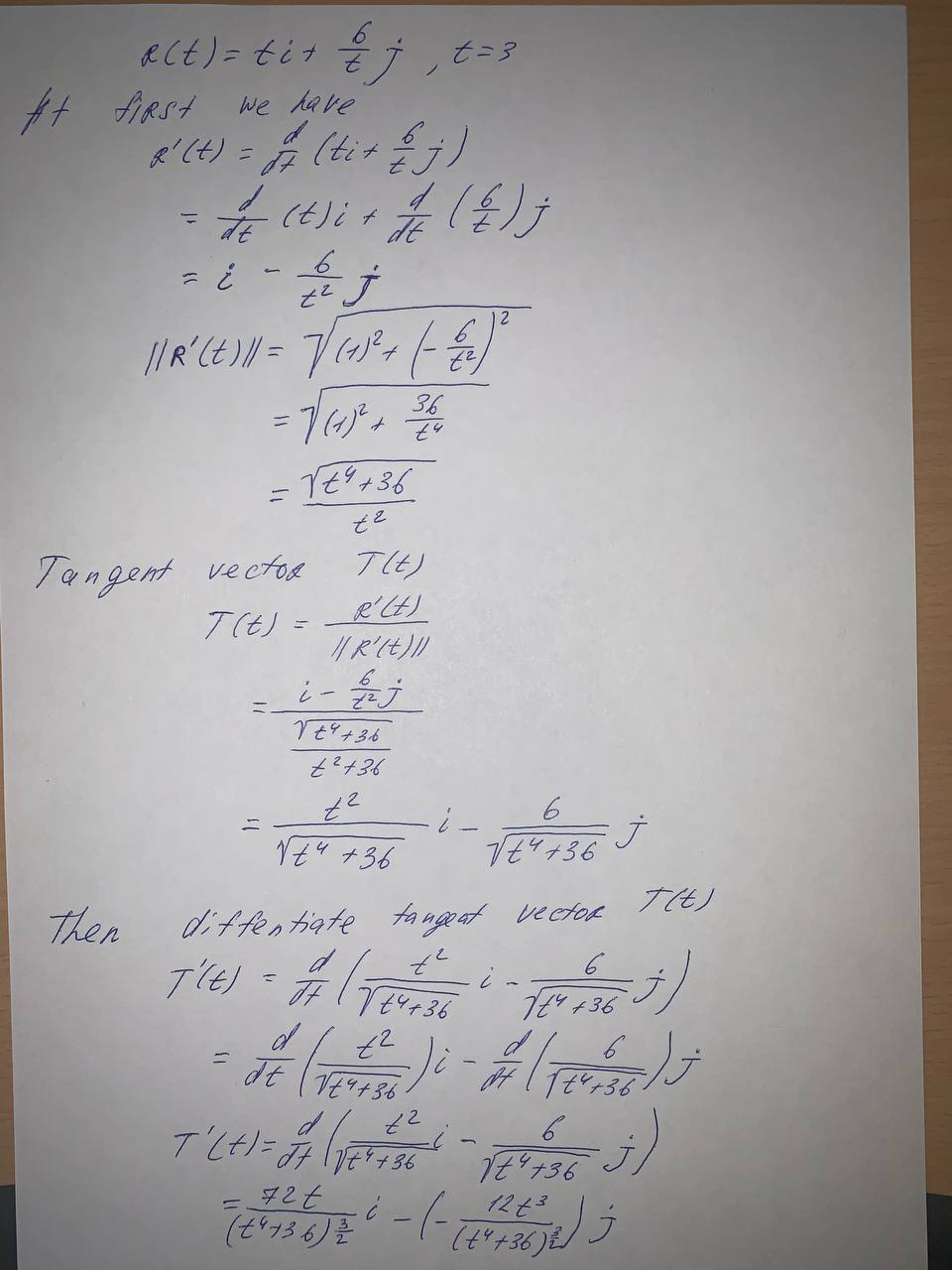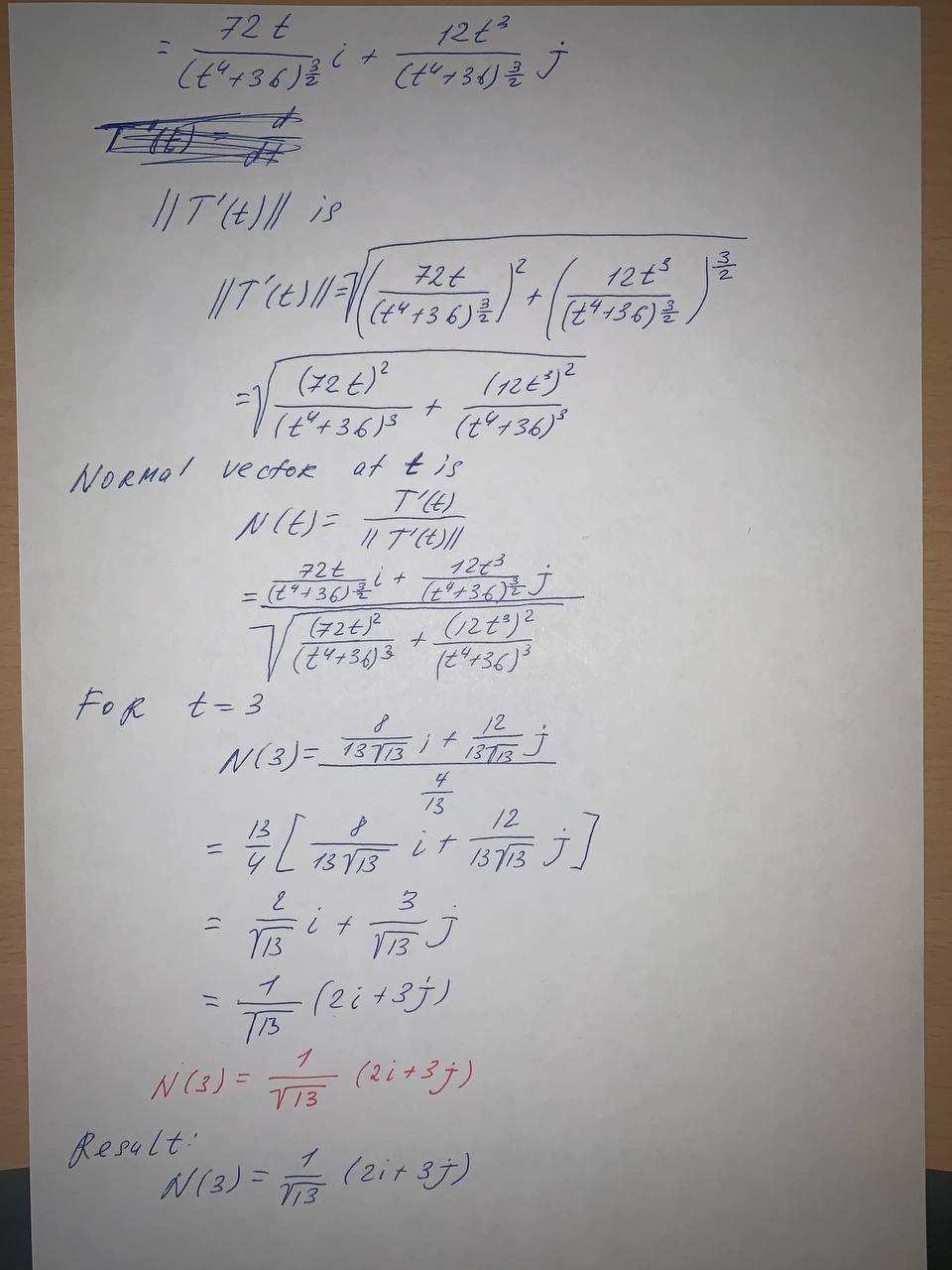Question# Find the principal unit normal vector to the curve at the specified value of the parameter r(t)=ti+\frac{6}{t}j , t=3

Vectors and spaces
ANSWEREDFind the principal unit normal vector to the curve at the specified value of the parameter
$$r(t)=ti+\frac{6}{t}j , t=3$$